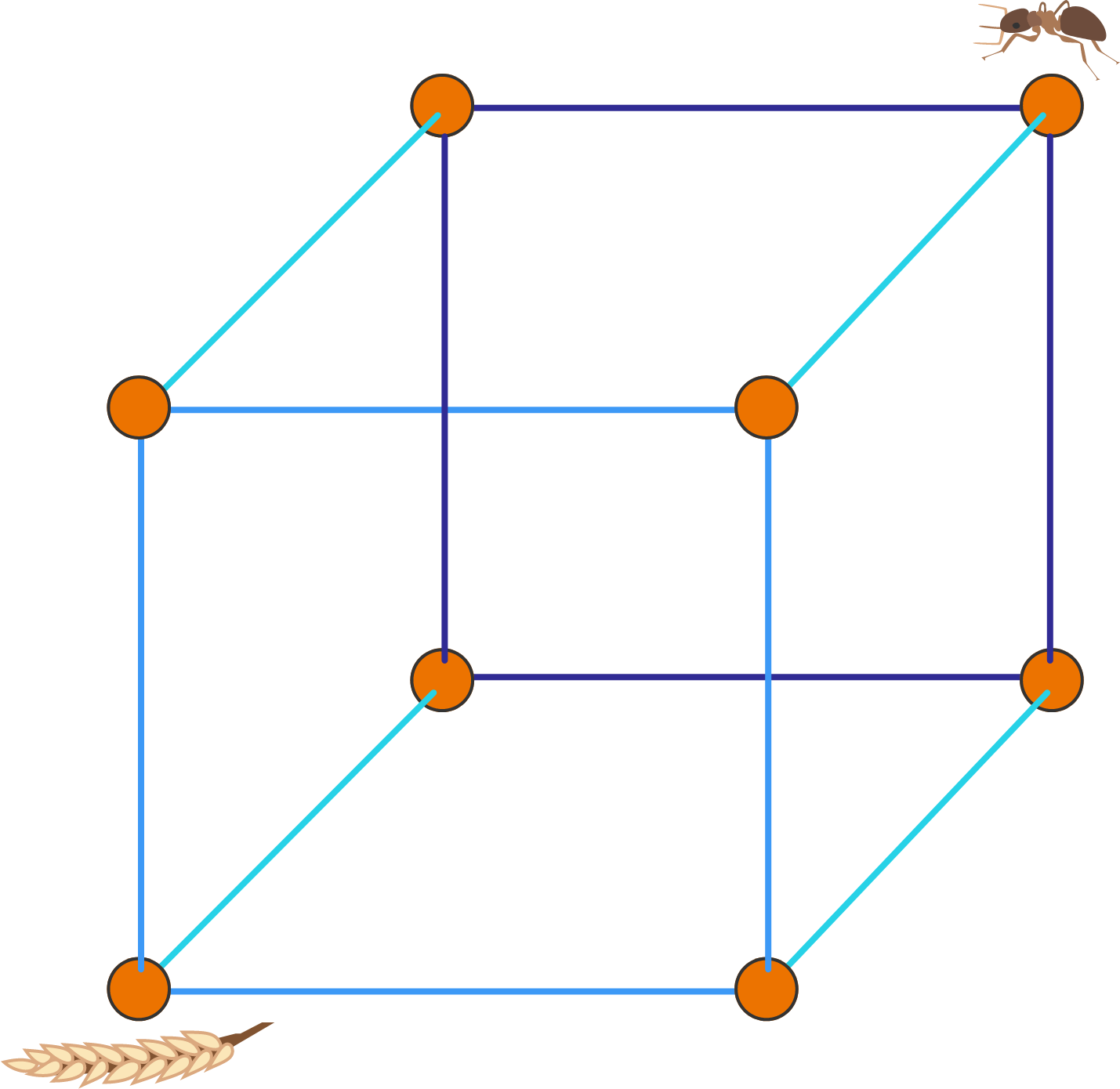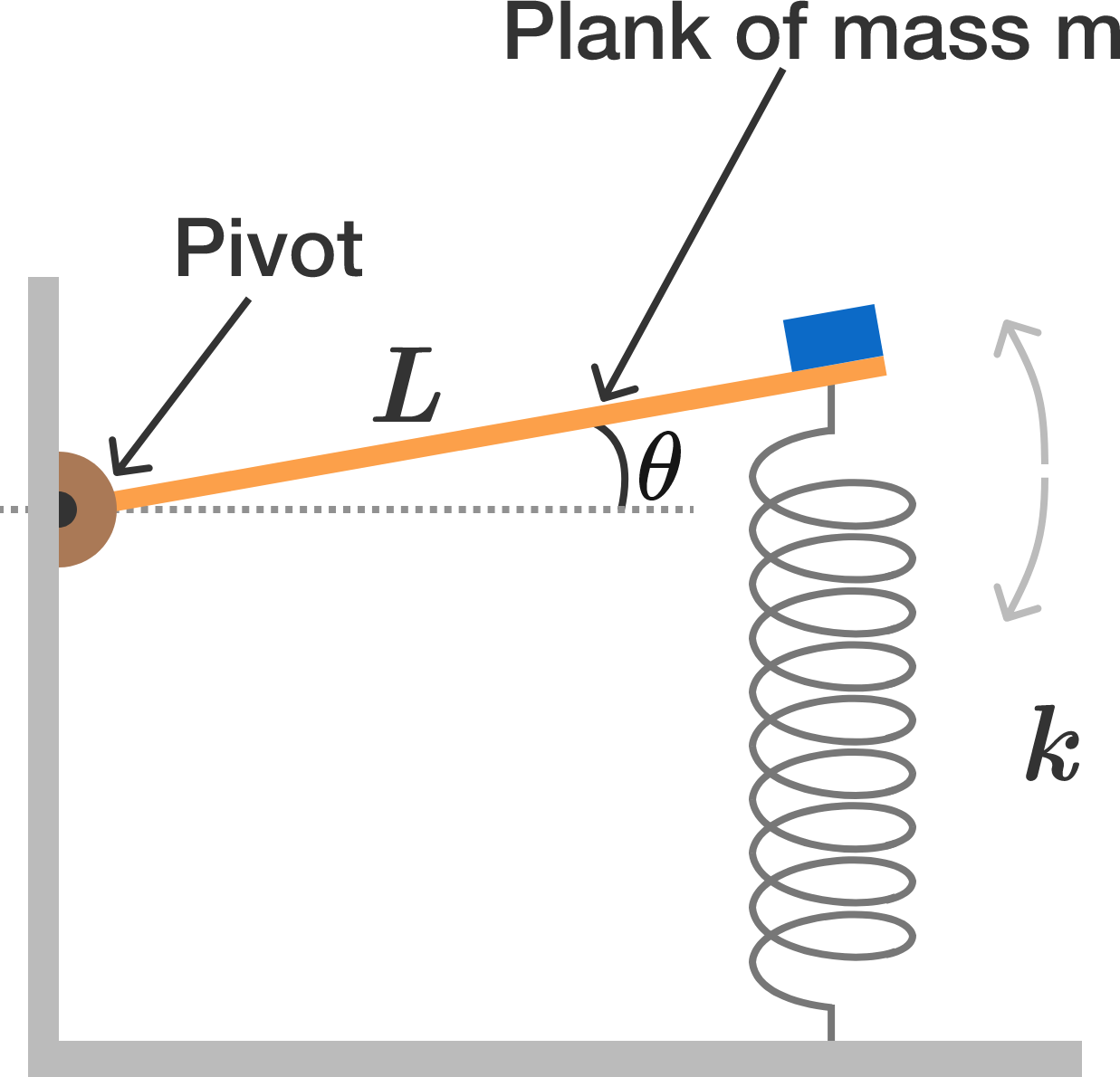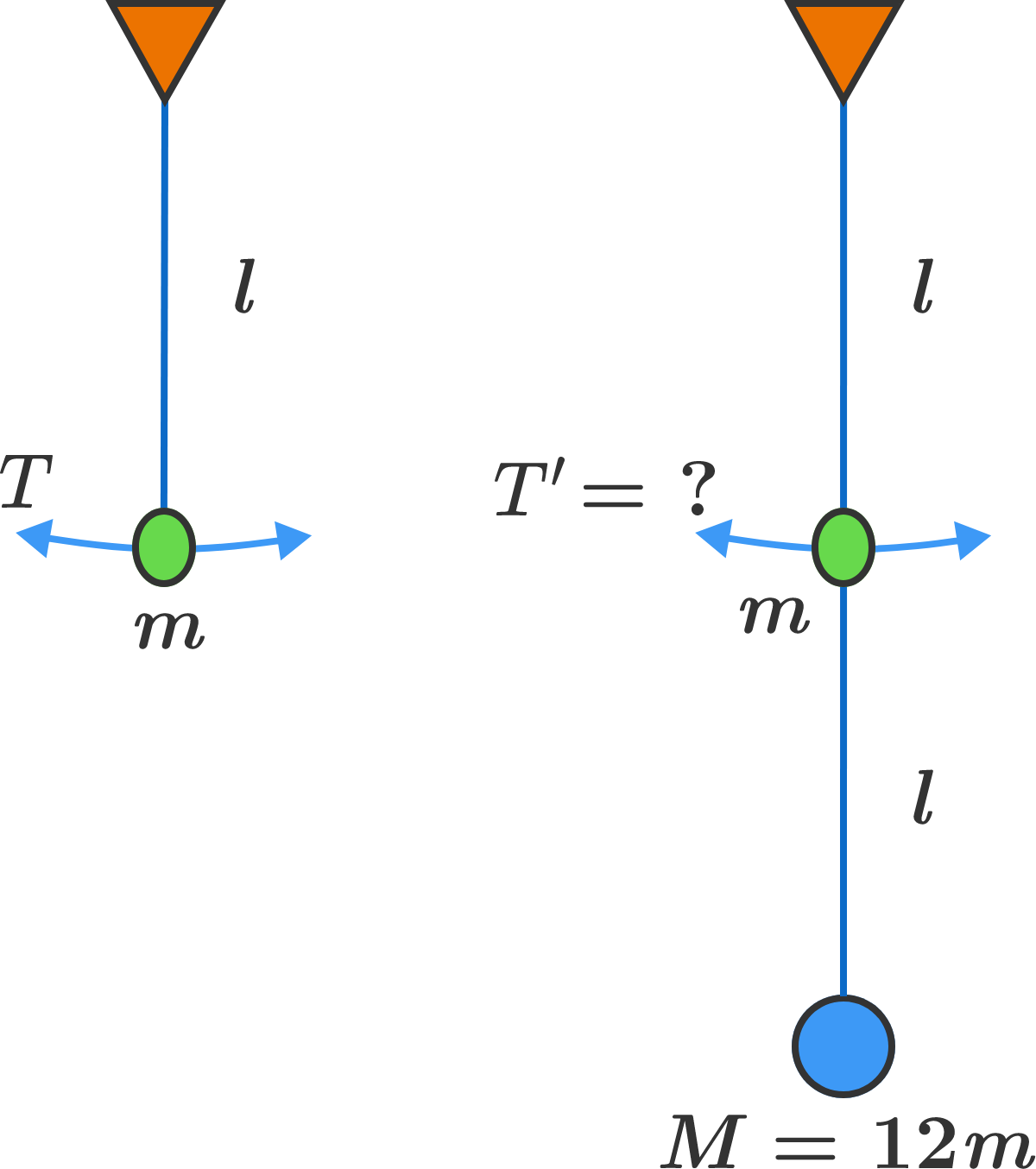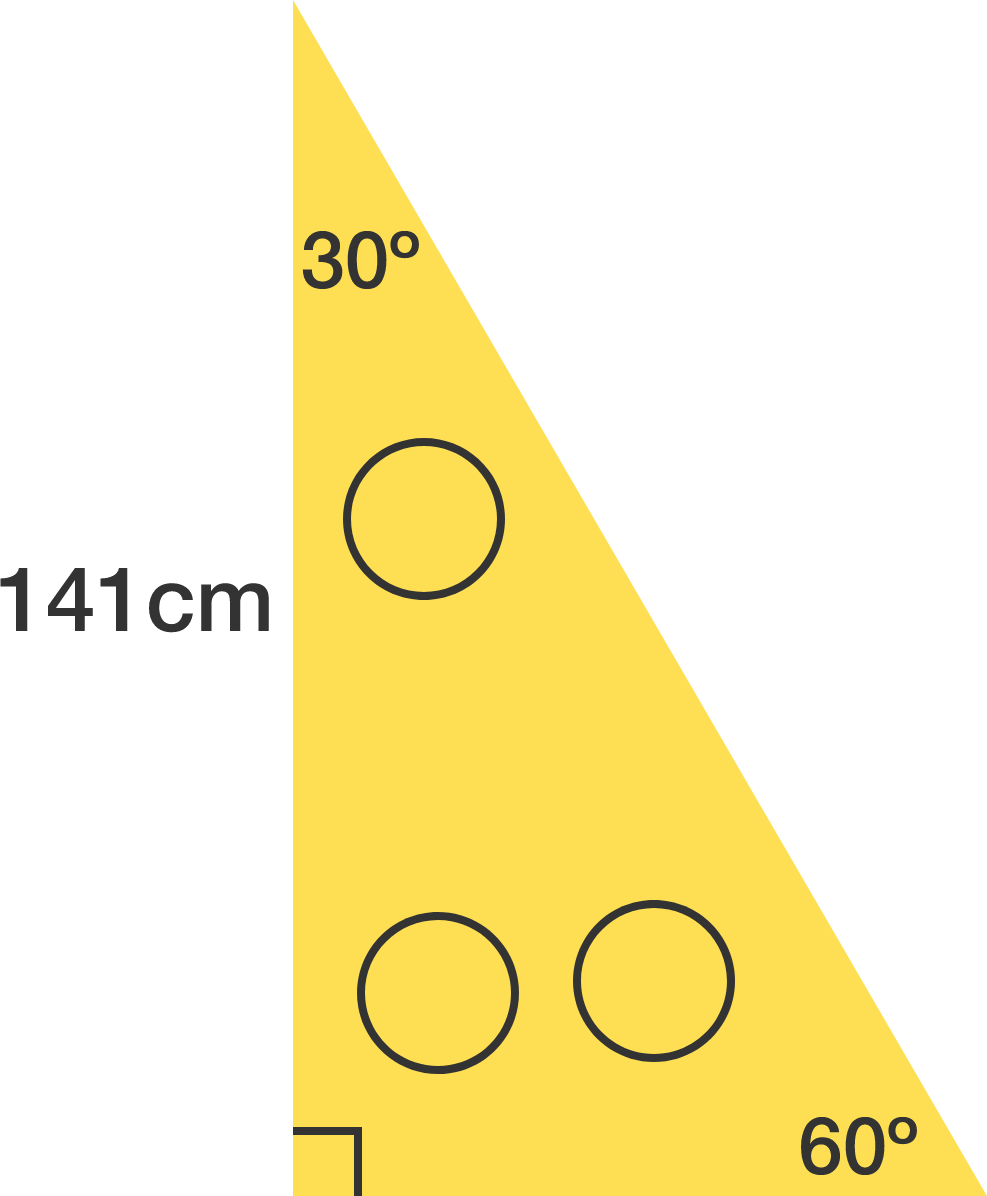# Problems of the Week

Contribute a problemThe ant, located on a vertex of a cube, wishes to reach the wheat at the opposite vertex of the cube. The ant selects an adjacent edge uniformly at random before moving along it. The ant continues moving in this manner, but it will never travel to a vertex it just arrived from.

What is the expected value of the number of moves for the ant to reach its destination?A horizontal plank of mass $m$ and length $L$ is pivoted at one end and is attached by a spring of force constant $k$ at the other end.

A small mass M is glued at the free end of the plank supported by the spring.

When the plank is displaced upward by a small angle of $\theta$ from its equilibrium position and released, it undergoes simple harmonic oscillations at angular frequency expressed as $\omega = \sqrt{\dfrac{ak}{m + bM}}.$ What is $a + b?$

A simple pendulum of length $\ell$ and bob mass $m$ undergoes small oscillations with the standard period $T = 2\pi\sqrt{\frac{\ell}g}.$

We now attach the second string of length $\ell$, with a bob of mass $12m,$ and hang it from the first bob. If we give a small kick to the bob of mass $m,$ initially the larger mass will hardly move while the small mass will oscillate with period $T^\prime$.

Find $\frac{T^\prime}T.$Note: The ropes are massless and flexible.The goal is to design an algorithm that will take the lists (12345678, XY) and convert them to (87654321, XY). The algorithm must move elements in the following manner:

• Select exactly two consecutive elements from the first list.
• Without changing their order, exchange those elements with the two elements in the second list.

What is the least number of moves required by the algorithm?

Hint: The 13 steps below, even though non-optimal, show how to accomplish the job following the rules. So, your answer must be smaller than 13.

[Step 00] 1 2 3 4 5 6 7 8 (X Y)
[Step 01] 1 2 3 4 5 6 X Y (7 8)
[Step 02] 7 8 3 4 5 6 X Y (1 2)
[Step 03] 7 1 2 4 5 6 X Y (8 3)
[Step 04] 8 3 2 4 5 6 X Y (7 1)
[Step 05] 8 7 1 4 5 6 X Y (3 2)
[Step 06] 8 7 1 4 5 3 2 Y (6 X)
[Step 07] 8 7 6 X 5 3 2 Y (1 4)
[Step 08] 8 7 6 X 1 4 2 Y (5 3)
[Step 09] 8 7 6 5 3 4 2 Y (X 1)
[Step 10] 8 7 6 5 3 X 1 Y (4 2)
[Step 11] 8 7 6 5 4 2 1 Y (3 X)
[Step 12] 8 7 6 5 4 3 X Y (2 1)
[Step 13] 8 7 6 5 4 3 2 1 (X Y)

Three identical circles are cut out from a 30$^\circ$-60$^\circ$-90$^\circ$ right triangle with the second largest side length 141 centimeters.

To three decimal places, what is the largest possible radius of these circles in centimeters?×